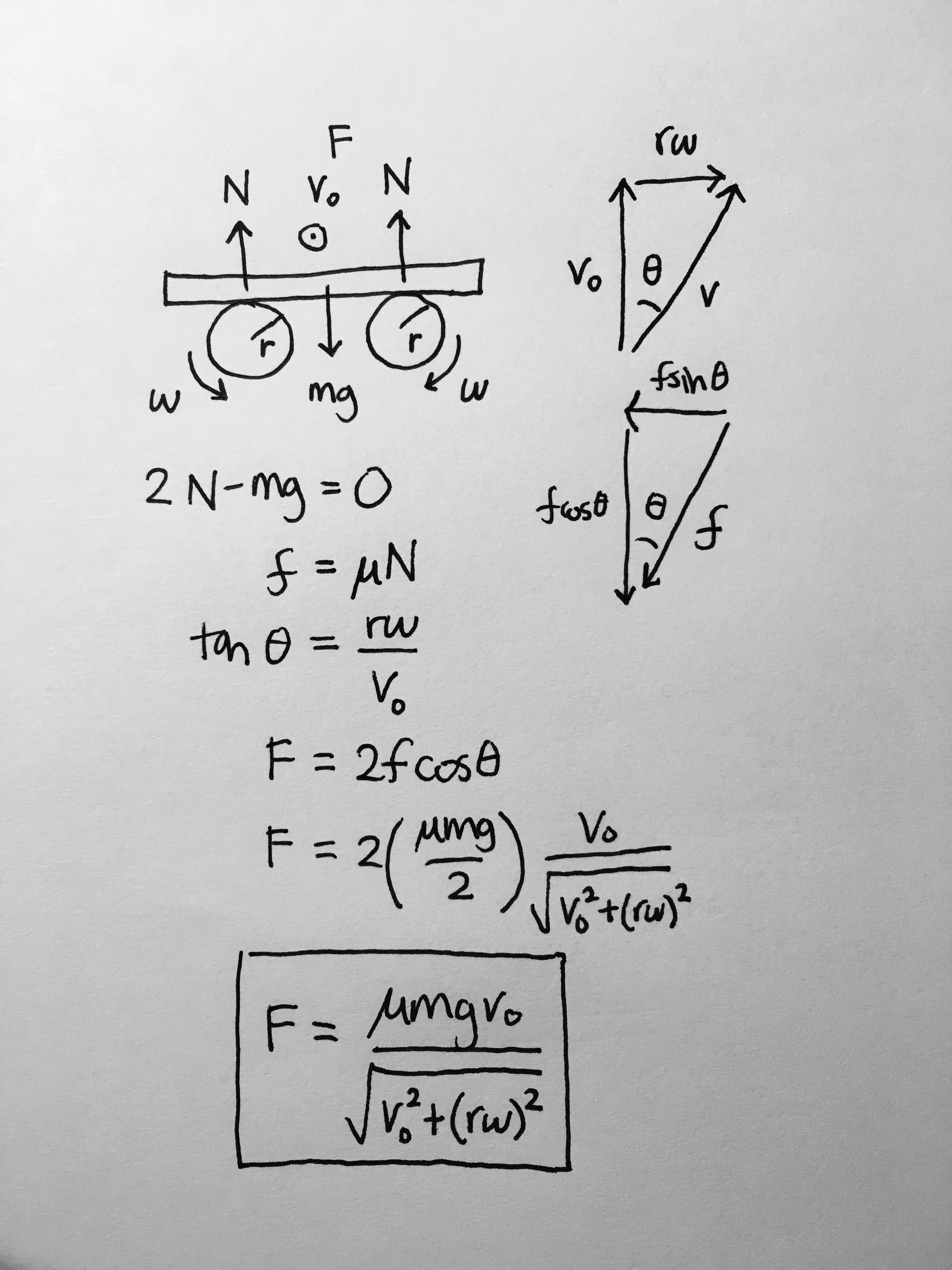# Statics

[started] 1/25/17

Friction

• Direction: Opposes relative motion between two surfaces
• Magnitude:
• Kinetic friction$f_k=\mu_k N$
• Static friction, matches external force up to maximum$f_s \leq \mu_s N$
• Concept: Friction points opposite to relative velocity
• Problem: A thin slab of mass$m$ rests centered on two cylinders of radius$r$ rotating with angular velocity$\omega$ in opposite directions. What force$F$ is required for the slab travel with velocity$v_0$ across the cylinders? The coefficient of friction is$\mu$.• Concept: Reaction force angle
• The reaction force$R$ is the net force of friction$f$ and the normal force$N$. The reaction angle$\varphi=\arctan(\frac{f}{N})$ is the angle between the reaction force and normal force. Once the interaction between two surfaces is determined, the reaction angle does not change.
• Problem: A force$F$ is applied at an angle$\theta$ from the hortizontal to move a block across the ground wih constant velocity. At what angle is the force minimized? The coefficient of friction is$\mu$• Concept: Self-locking
• Occurs when the applied force$F$ to displace an object contributes to the normal force$N$ and friction$f$. There is a critical angle$\theta$ beyond which the object will not move no matter how much force is applied.
• Problem: A rod of length$l$ rests vertically on the ground. A rope is attached to the top end and the ground, making an angle$\theta$ with the verticle. If a force$F$ is applied horizontally on the rod, how far from the bottom does it need applied to be for the rod to self-lock? The coefficient of friction is$\mu_0$.